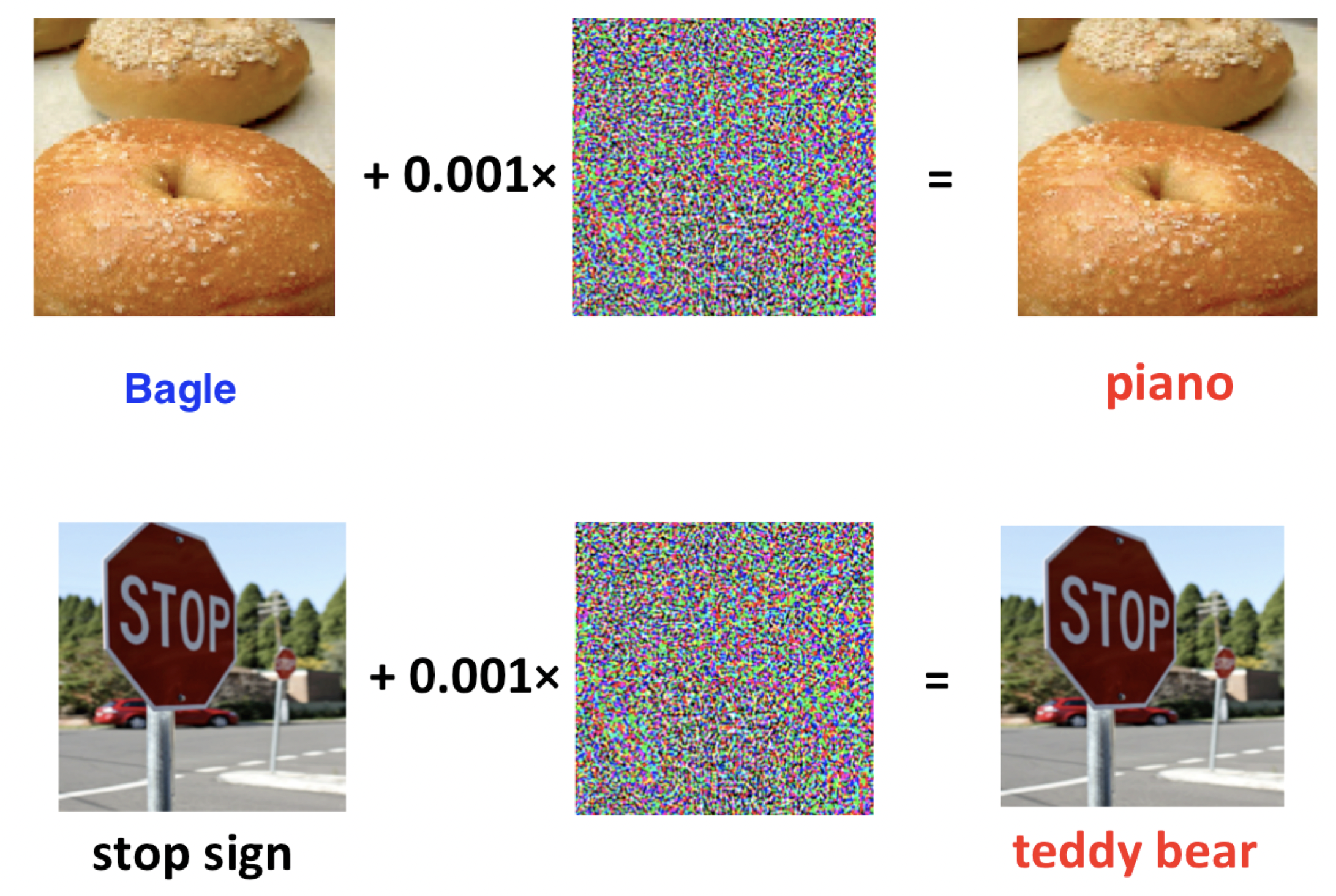# Lipschitz constant of a convolutional layer in neural network

It was observed, that small perturbation in Neural Network input could lead to significant errors, i.e. misclassifications.Lipschitz constant bounds the magnitude of the output of a function, so it cannot change drastically with a slight change in the input

$\|NN(image) - NN(image+\varepsilon)\| \leq L\|\varepsilon\|$

In this notebook we will try to estimate Lipschitz constant of some convolutional layer of a Neural Network.